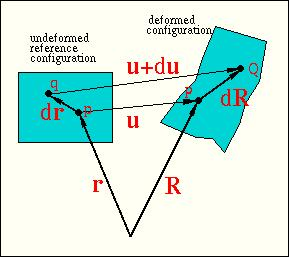# Postgraduate Lecture: Nonlinear Elasticity and Computational Solid Mechanics

### Fridays 4-5pm Lecture Theatre G.06

The course is mainly aimed at Applied/NA postgraduates (MSc and PhD) for whom attendance is compulsory. All other students and members of staff are also most welcome to attend. The course is taught by Dr. Matthias Heil. Note that no prior knowledge of continuum mechanics will be required.

The motivation for the course is as follows: The nonlinear Navier Stokes equations of fluid mechanics are well known in their exact form and are commonly used as the starting point for any mathematical investigation. Linearisations about specific reference states can easily be obtained.

Conversely, most of the elasticity literature (and indeed the department's elasticity course MT4721) is based on linearised theories and is therefore a priori restricted to infinitesimal displacements/deformations. Attempts to extend the linearised theory to larger displacements are tedious (and error prone!) since many of the complications associated with large displacements cannot really be appreciated from the framework of a small displacement-theory. In many ways it is easier to re-derive the governing equations of continuum mechanics (without any restrictions on the magnitude of the displacements) from scratch. In this framework, linear and weakly non-linear theories appear as subset of the general theory. The lecture course will follow this approach.The outline of the course is as follows:

• After an introduction which will provide examples and applications of large-displacement (nonlinear) elasticity, we will begin with a brief review of tensor analysis and derive a strain measure that is suitable to describe large deformations (Green's strain tensor).

• Next we will investigate various large-displacement stress measures and derive the corresponding equilibrium equations. Constitutive equations (i.e. the stress-strain relationships) will be discussed briefly.

• Unsurprisingly, the governing equations will turn out to be fairly complicated (even for the purposes of a numerical solution). A more convenient formulation which is also ideally suited for a Finite Element discretisation is given by the `Principle of Virtual Displacements' which we will discuss in some detail.

• Finally, techniques for the numerical solution of large displacement elasticity problems will be discussed and the solution strategy will be illustrated by a detailed example.

This will be followed by a visit to a local pub...

If you have any questions about the lecture, please see me in my office (18.07), contact me by email ( M.Heil@maths.man.ac.uk) or catch me after the lecture.